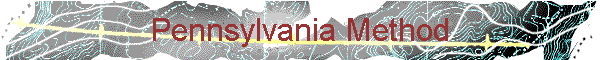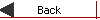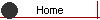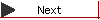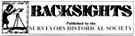Article taken from "Backsights" Magazine published by Surveyors Historical Society

THE PENNSYLVANIA METHOD

by Wilhelm A. Schmidt, PLS

The measure of land is its area. But area itself is not measured. It is calculated.

The calculation of the area of a piece of land is easy enough when it has a regular shape. A regularly shaped piece of land, of course, has many practical advantages. Towns, cities, counties and large portions of this country are laid out in a grid, not merely for aesthetic reasons or for ease of laying them out, but because a grid allows for an eminently efficient use of the land. But for surveyors and assessors, the advantage of the regular shape, especially of small lots, is that it makes area calculations a matter of simple multiplication.

Unfortunately, parcels of land are seldom regular in shape. Often, especially in colonial days, they were occupied long before they were surveyed. Inhabitation followed the terrain, an invariable feature of which is its irregularity. The resulting pieces of ground usually had straight lines between corners, but indeterminate shapes.

Calculating the area of such a form has been known since Euclid. The trick is to break up the irregular shape into manageable components, and then perform the appropriate multiplications and sums. By colonial times, the mathematics involved were greatly facilitated by the invention of logarithms and trigonometric function tables. Given these, and reasonably accurate field measurements, any colonial surveyor could calculate its area with a pencil - "more or less".

But it was an arduous task - and still was, more than forty years ago, when I landed my first surveying summer job. By then, rotary and electrified calculators helped, but the many steps it took still had to be performed one by one. The steps became programmed with the invention of computers, and require a mere push of a key or click of the mouse to solve an area. Oh, for the good old days! At least, we knew how it was done.

The colonial surveyors were using a standardized method called "DMD", the abbreviation for double mean distance or double meridian distance.

Once transits were used, the first step in the DMD method was to balance the angles. They could be interior or exterior. The field check was to add up the number of sides to the figure, either less by two (interior) or more by two (exterior), times 180 degrees. That sum could be compared to the sum of the angles turned for an initial check. The angles were not always adjusted for balance, but if they were, the error was most likely distributed proportionately among all the angles.

The second step was to calculate the latitudes and longitudes. This required looking up the cosines and sines for the directions of the property lines with respect to north and south. (This is the rationale for quadrants in the first place). These functions were generally carried to eight places after the decimal point, and then multiplied by the lengths of the lines. The results were arranged in columns: N, S, E, and W. North and East were positive, and South and West were negative.

The third step was to balance these columns. Theoretically, the sum of the latitudes and the sums of the departures must equal zero, for the figure to close. Since measurements are inherently imprecise, they never really equalled zero, and were mildly suspect when they did. From the difference in the starting and ending latitudes and departures, the direction and length of the closing line were calculated. The error of closure was most easily eliminated by placing it in the line(s) whose direction most nearly mimicked the closing line. The error could also be distributed in other ways. The most methodical way was to distribute the error proportionately, the correction in each line being determined by the closing line multiplied by the ratio of a line length to the total perimeter length. However the error was reapportioned, the latitudes and departures would then be recalculated.

The next step was to calculate a series of areas, one corresponding to each of the parcel lines. A longitudinal line was drawn, at least hypothetically, through the westerly-most corner of the parcel plat, and latitudinal lines drawn from the corners to that line. The result was a series of areas, the first and last of which were triangular and the rest trapezoidal in shape. At this point, the procedure was to add the two departures of each area and multiply the sum by the longitudinal divergence of the line. The result was not an absolute number for each area, but a number with a positive or negative sign, derived from the signs ascribed in step two.

The last step was to add all these areas and divide the sum by two. By simply adding the two sides of each trapezoidal area, a rectangular area double the size of the trapezoid was calculated, thus requiring the division by two.

Whew! There you have it. But it takes so long. Isn’t there a simpler way?

There is, although not much simpler. It is called the Pennsylvania method, attributed to David Rittenhouse (1732-1796). Since the area of a trapezoid is obtained by multiplying the average of the two parallel sides by its height - he must have reasoned - why not divide each sum of the sides by two? And why not add the sum of the longitudes and the sum of the departures (not forgetting their signs) and multiply these sums? The answer is the same, and the method acquired a regional name.